# Pre-Algebra : Identities and Properties

## Example Questions

← Previous 1 3 4 5 6 7 8 9 10

### Example Question #361 : Operations And Properties

What property can be applied to the following expression?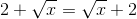Commutative of Multiplication

Explanation:

The rule for Commutative Property of Addition is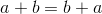Expression given in the question is:Hence the property is Commutative of Addition.

### Example Question #1 : Commutative Property Of Addition

Identify the property being applied.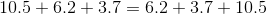Order of Operations

Associative Inverse Property

Explanation:

This property states that any set of terms can be added together in any order to achieve the same solution.

### Example Question #3 : Identities And Properties

Which of the following statements demonstrates the commutative property of addition?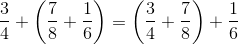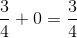None of the examples in the other responses demonstrates the commutative property of addition.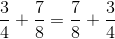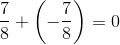Explanation:

The commutative property of addition states that two numbers can be added in either order to obtain the same sum. Of the given responses, onlydemonstrates this property, so it is the correct choice.

### Example Question #371 : Operations And Properties

Which of the following best represents the commutative property of addition?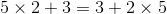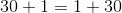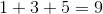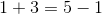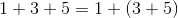Explanation:

The property is defined as: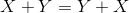Switching the order of the numbers and adding the numbers will yield the same result.

The only answer is:### Example Question #1 : Identities And Properties

Which of the following choices best represent the commutative property of addition?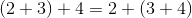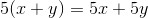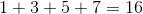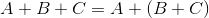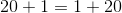Explanation:

Write the rule for the commutative property of addition, lettingandbe any number.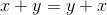Changing the order of these two numbers will not affect the final answer.

The best choice is:### Example Question #1 : Commutative Property Of Multiplication

Which property is illustrated by the example: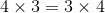Associative property of multiplication

Multiplicative identity property

Commutative property of multiplication

Distributive property

Commutative property of multiplication

Explanation:

The commutative property of mulitplication states that the order in which 2 numbers are multiplied does not affect the result: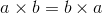Or in this case,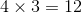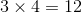### Example Question #2 : Commutative Property Of Multiplication

Use the Commutative Property of Multiplication to write the below expression in a different way.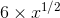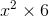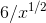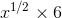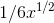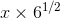Explanation:

The rule for Commutative Property of Multiplication isUsing this rule, the expressioncan be written as### Example Question #3 : Commutative Property Of Multiplication

Which of the following equations correctly shows the commutative property of multiplication?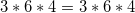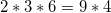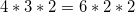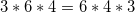Explanation:

The commutative property states that factors can be multiplied in any order and the product is always the same. While each of our answer choices are real equations, only one correctly displays this property: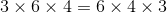### Example Question #1 : Commutative Property Of Multiplication

Evaluate the following expression without using a calculator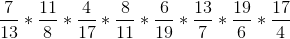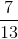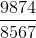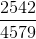Explanation:

This problem could easily turn into some super heavy calculations, but nobody has time for that.  Instead, we can make this problem very easy by utilizing the commutative property of multiplication, which in essence says that numbers can be multiplied in any order.

In other words,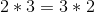and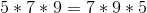That means that rather multiplying our numbers in their orginal order, which can actually change the order of the numbers without affecting the final product.  At this point we should notice that one of our numbers iswhile another is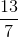.  Similarly the fractions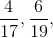and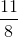are each accompanied by their recripocals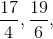and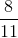respecitively.  At this point we must also remember the multiplicative inverse property, which tells us that the product of a number and its reciprocal will always be 1.  With that in mind, the strategic way to order the multiplication is as follows: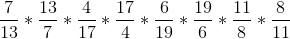Doing this pairs each number up with its reciprocal.  Finally, we can utlize the associative properpty of multiplication, which tells us that we can multiply any two numbers at a time.

In other words,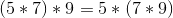.  This allows us to pair off the multiplication as follows.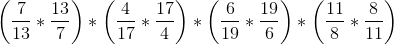Within each parentheses we now have a number multiplied by its reciprocal.  Remembering that this always equals 1, we can simplify, giving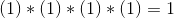### Example Question #5 : Commutative Property Of Multiplication

Which answer shows the communitive property of multiplication to the equation below?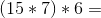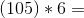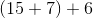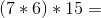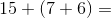Explanation:

The communitive property of multiplication is a property that states that the numbers can be multiplied in any combination. To show which answer is correct simply regroup the numbers in a different or and you will have your answer.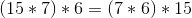← Previous 1 3 4 5 6 7 8 9 10

### All Pre-Algebra Resources